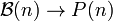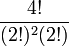# Combinatorics of symmetric group:S4

## Contents

View combinatorics of particular groups | View other specific information about symmetric group:S4

This page discusses some of the combinatorics associated with symmetric group:S4, that relies specifically on viewing it as a symmetric group on a finite set. For more on the element structure from a group-theoretic perspective, see element structure of symmetric group:S4.

## Partitions, subset partitions, and cycle decompositions

Denote by$\mathcal{B}(n)$ the set of all unordered set partitions of$\{ 1,2,\dots,n\}$ into subsets and by$P(n)$ the set of unordered integer partitions of$n$. There are natural combinatorial maps:$S_n \to \mathcal{B}(n) \to P(n)$

where the first map sends a permutation to the subset partition induced by its cycle decomposition, which is equivalently the decomposition into orbits for the action of the cyclic subgroup generated by that permutation on$\{ 1,2,\dots,n\}$. The second map sends a subset partition to the partition of$n$ given by the sizes of the parts. The composite of the two maps is termed the cycle type, and classifies conjugacy classes in$S_n$, because cycle type determines conjugacy class.

Further, if we define actions as follows:

•$S_n$ acts on itself by conjugation
•$S_n$ acts on$\mathcal{B}(n)$ by moving around the elements and hence changing the subsets
•$S_n$ acts on$P(n)$ trivially

then the maps above are$S_n$-equivariant, i.e., they commute with the$S_n$-action. Moreover, the action on$\mathcal{B}(n)$ is transitive on each fiber above$P(n)$ and the action on$S_n$ is transitive on each fiber above the composite map to$P(n)$. In particular, for two elements of$\mathcal{B}(n)$ that map to the same element of$P(n)$, the fibers above them in$S_n$ have the same size.

There are formulas for calculating the sizes of the fibers at each level.

In our case$n = 4$:

Partition Partition in grouped form Formula calculating size of fiber for$\mathcal{B}(n) \to P(n)$ Size of fiber for$\mathcal{B}(n) \to P(n)$ Formula calculating size of fiber for$S_n \to \mathcal{B}(n)$ Size of fiber for$S_n \to \mathcal{B}(n)$ Formula calculating size of fiber for$S_n \to P(n)$, which is precisely the conjugacy class size for that cycle type (product of previous two formulas) Size of fiber for$S_n \to P(n)$, which is precisely the conjugacy class size for that cycle type (product of previous two sizes)
1 + 1 + 1 + 1 1 (4 times)$\frac{4!}{(1!)^44!}$ 1$(1 - 1)!^4$ 1$\frac{4!}{1^44!}$ 1
2 + 1 + 1 2 (1 time), 1 (2 times)$\frac{4!}{(2!)(1!)^2(2!)}$ 6$(2 - 1)!(1 - 1)!^2$ 1$\frac{4!}{(2)(1)^2(2!)}$ 6
2 + 2 2 (2 times)$\frac{4!}{(2!)^2(2!)}$ 3$(2 - 1)!^2$ 1$\frac{4!}{(2)^2(2!)}$ 3
3 + 1 3 (1 time), 1 (1 time)$\frac{4!}{(3!)^1(1!)(1!)^1(1!)}$ 4$(3 - 1)!(1 - 1)!$ 2$\frac{4!}{(3)^1(1!)(1^1)(1!)}$ 8
4 4 (1 time)$\frac{4!}{(4!)^1(1!)}$ 1$(4 - 1)!$ 6$\frac{4!}{(4^1(1!)}$ 6
Total (5 rows -- number of rows equals number of unordered integer partitions of 4) -- -- 15 (equals the size of$\mathcal{B}(n)$, termed the Bell number, for$n = 4$) -- 11 (see Oeis:A107107) -- 24 (equals 4!, the size of the symmetric group)